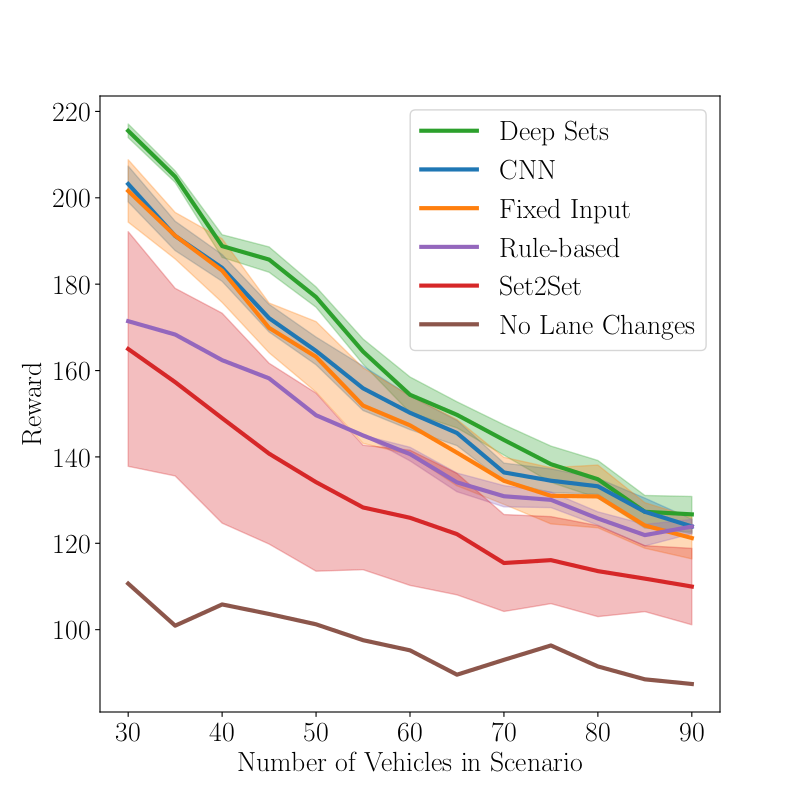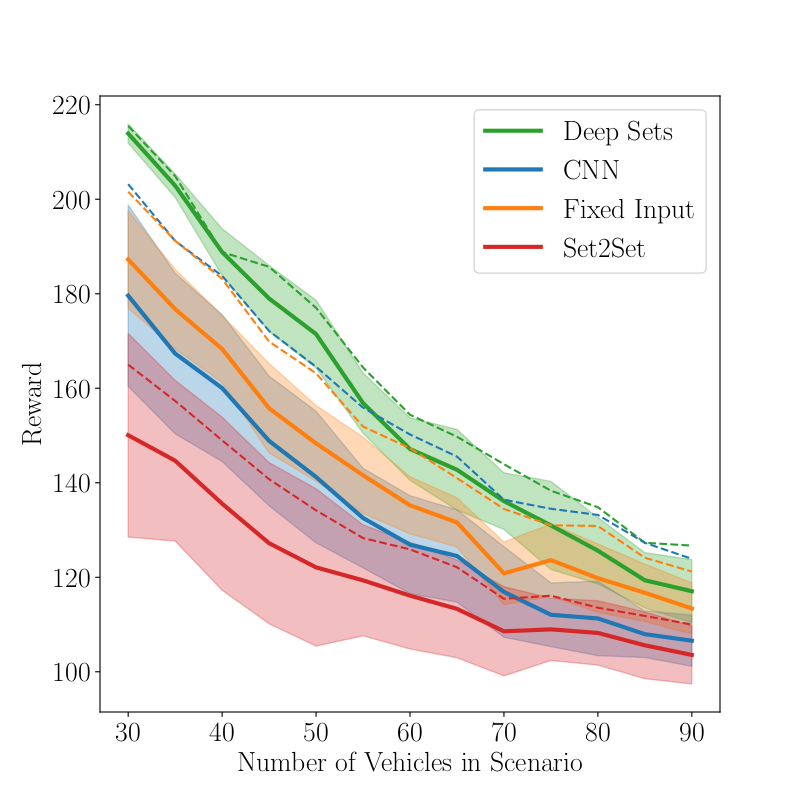In this work, we elaborate limitations of fully-connected neural networks and other established approaches like convolutional and recurrent neural networks in the context of reinforcement learning problems that have to deal with variable sized inputs. We employ the structure of Deep Sets in off-policy reinforcement learning for high-level decision making, highlight their capabilities to alleviate these limitations, and show that Deep Sets not only yield the best overall performance but also offer better generalization to unseen situations than the other approaches.

## Publication

Maria Huegle, Gabriel Kalweit, Branka Mirchevska, Moritz Werling and Joschka Boedecker (2019). Dynamic Input for Deep Reinforcement Learning in Autonomous Driving. In IEEE/RSJ International Conference on Intelligent Robots and Systems (IROS).Fig. 1: Limitations of fixed input representations.

## Motivation

Prior research mostly relied on fixed sized inputs or occupancy grid representations for value or policy estimation. However, fixed sized inputs limit the number of considered vehicles, for example to the $n$-nearest vehicles. Fig. 1 (a) shows a scenario with a fixed input representation of the $n=2$ nearest surrounding vehicles, where vehicles 3 and 4 are not visible. Cars considered by the input representation are shown in white, invisible cars in red and the agent in blue. This kind of representation is not enough to make the optimal lane change decision, since the agent does not represent the $n+1$ and $n+2$ closest cars even though they are within sensor range.

A more advanced fixed sized representation considers a relational grid of $\Delta_{\text{ahead}}$ leaders and $\Delta_{\text{behind}}$ followers in $\Delta_{\text{lateral}}$ side lanes around the agent. As Fig. 1 (b) shows, for a relational grid with $\Delta_{\text{lateral}} = 1$ considered side lanes, this can still be insufficient for optimal decision making, since the white car on the incoming $\Delta_{\text{lateral}}+1$ right lane is not within the maximum of considered lanes, and therefore has no influence on the decision making process.

## Technical ApproachFig. 2: Scheme of DeepSet-Q algorithm.

We train a neural network $Q_{\mathcal{DS}}(\cdot, \cdot|\theta^{Q_{\mathcal{DS}}})$, parameterized by $\theta^{Q_{\mathcal{DS}}}$, to estimate the action-value function via DQN. The state consists of dynamic input $X_t^{\text{dyn}}=[x^1_t, .., x^{\text{seq len}}_t]^\top$ with a variable number of vectors $x^j_t|_{0\le j \le \text{seq len}}$ and a static input vector $x_{t}^{\text{static}}$. In the application of autonomous driving, the sequence length is equal to the number of vehicles in sensor range. The Q-network $Q_{\mathcal{DS}}$ consists of three main parts, $(\phi,\rho,Q)$. The input layers are built of two neural networks $\phi$ and $\rho$, which are components of the Deep Sets. The representation of the input set is computed by: $$\Psi(X_t^{\text{dyn}}) = \rho\left(\sum_{x^j_t\in X_t^{\text{dyn}}}\phi(x_t^j)\right),$$ which makes the Q-function permutation invariant w.r.t. its input. An overview of the Q-function is shown in Fig. 2, where the static feature representations $x_t^{\text{static}}$ are fed directly to the $Q$-module. The final Q-values are then given by $Q_{\mathcal{DS}}(s_t, a_t)=Q([\Psi(X_t^{\text{dyn}}), x_{t}^{\text{static}}], a_t)$ for action $a_t$. We then minimize the loss function: $$L(\theta^{Q_{\mathcal{DS}}}) = \frac{1}{b}\sum\limits_i\left(y_i - Q_{\mathcal{DS}}(s_i, a_i)\right)^2,$$ with targets $y_i=r_i + \gamma \max_{a} Q'_{\mathcal{DS}}(s_{i+1}, a),$ where $(s_i, a_i, s_{i+1}, r_i)|_{0\leq i \leq b}$ is a randomly sampled minibatch from the replay buffer. The target network is a slowly updated copy of the Q-network. In every time step, the parameters of the target network $\theta^{Q'_{\mathcal{DS}}}$ are moved towards the parameters of the Q-network by step-length $\tau$, i.e. $\theta^{Q'_{\mathcal{DS}}}\leftarrow\tau\theta^{Q_{\mathcal{DS}}} + (1-\tau)\theta^{Q'_{\mathcal{DS}}}$.

## Results

The Deep Set architecture yields the best performance and lowest variance across traffic scenarios in comparison to rule-based agents and reinforcement learning agents using Set2Set, fully-connected or convolutional neural networks as input modules. The results are depicted in Fig. 3. The differences between the approaches get smaller as more vehicles are on the track due to general maneuvering limitations in dense traffic.

To investigate the generalization capabilities of all methods we trained additionally transitions of at most six surrounding vehicles (see Fig. 4). This is only a small fraction of possible traffic situations. CNNs have difficulties generalizing to unseen situations and suffer from larger variance in comparison to training on the full data set. In contrast, Deep Sets are able to mostly keep the performance even when trained on the truncated dataset, showing only a small increase in variance. Additionally, we evaluated generalization cabilities for more lanes and sensor noise. Details are shown in the paper.Fig. 3Fig. 4
Results for the DeepSet-Q Algorithm in the Highway scenario when (Fig. 3) trained on the full data set and (Fig. 4) trained on transitions with at most 6 vehicles.

## Demonstration

Video explaining methods and performance of DeepScene-Q in the SUMO traffic simulator.

### BibTeX


@misc{huegle2019dynamic,
title={Dynamic Input for Deep Reinforcement Learning in Autonomous Driving},
author={Maria Huegle and Gabriel Kalweit and Branka Mirchevska and Moritz Werling and Joschka Boedecker},
year={2019},
eprint={1907.10994},
archivePrefix={arXiv},
primaryClass={cs.LG}
}


## People#### Maria Hügle

Website#### Gabriel Kalweit

Website#### Joschka Bödecker

Website